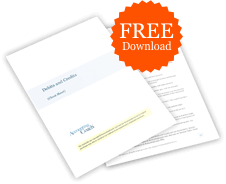# Kindly illustrate various depreciation methods.

## Definition of Depreciation

Depreciation is the systematic allocation of the cost of an asset to Depreciation Expenses over the asset's useful life.

If an asset will have a salvage value at the end of the asset's useful life, the amount of the salvage value is not depreciated. However, it is common to assume that the salvage value will be \$0.

The depreciation method used for financial reporting is often different from the depreciation method used for U.S. income tax reporting.

## Examples of Depreciation Methods

For our comparison of some of the depreciation methods, assume that a company purchased a new plant asset at a cost of \$100,000 that will have an estimated salvage value of \$10,000 at the end of its useful life. This means that the total amount of depreciation will be \$90,000 (which is referred to as the depreciable cost). The asset is expected to be useful for 5 years or the production of 100,000 units of output. The asset is placed into service on January 1, 2021, and the company's accounting year is January 1 through December 31.

## Straight-Line Method

Depreciable cost of \$90,000 divided by 5 years = \$18,000 of depreciation each year for 5 years. Download our Straight-line Method Form and Template.

## Units-of-Production Method

Depreciable cost of \$90,000 divided by 100,000 units = \$0.90 per unit. In 2021, the company produces 12,000 units X \$0.90 = \$10,800 of depreciation. In 2022, the company produces 30,000 units X \$0.90 = \$27,000 of depreciation. Continue until the accumulated depreciation reaches \$90,000. Download our Units-of-Activity (Production) Method Form and Template.

## Double-Declining-Balance Method

The straight-line depreciation rate is 20% (100% divided by 5 years). Double the straight-line rate = 40% (20% X 2). This rate is applied to the book value of the asset at the beginning of each year. (Book value is the asset's cost minus its accumulated depreciation.) For the year 2021, the double-declining-balance depreciation is: beginning book value of \$100,000 X 40% = \$40,000. For the year 2022, the calculation is: beginning book value of \$60,000 X 40% = \$24,000. Continue until the accumulated depreciation reaches \$90,000. Download our Double-Declining-Balance Method Form and Template.

## Sum-of-the-Years'-Digits Method

Add the digits in the years of useful life: 5+4+3+2+1 = 15. In the first year (2021), multiply 5/15 times the depreciable cost of \$90,000 = \$30,000 of depreciation. In 2022, multiply 4/15 times \$90,000 = \$24,000. In 2023, multiply 3/15 times \$90,000, and so on.### Free Debits and Credits Cheat Sheet

546,134
Subscribers
You are already subscribed. This offer is not available to existing subscribers.
Error: You have unsubscribed from this list.